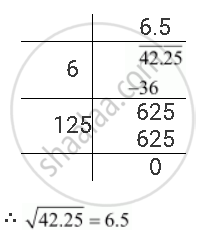Share

# Find the Square Root of the Following Decimal Number 42.25 - Mathematics

Course
ConceptSquare Roots of Decimals

#### Question

Find the square root of the following decimal number

42.25

#### Solution

The square root of 42.25 can be calculated as follows.Is there an error in this question or solution?

#### APPEARS IN

NCERT Solution for Mathematics Textbook for Class 8 (2018 to Current)
Chapter 6: Squares and Square Roots
Ex. 6.4 | Q: 3.4 | Page no. 108
Solution Find the Square Root of the Following Decimal Number 42.25 Concept: Square Roots of Decimals.
S